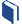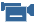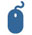# Fan Appreciation Night, Part 1

###Resources for this lesson:

> Glossary> Calculator Resources> Teacher Resources: Instructional Notes##Real-Life Scenarios

> Text version for animationLet’s explore the question:

If Khalid has an 80% chance of answering each question correctly, what is the probability that he would answer all 8 history questions correctly?

##Check Your Understanding

Let’s explore how to find this probability.

When presented with a situation, there are some general steps you can follow to solve the problem.

1. Create and Analyze – Look at the scenario and find various ways to illustrate the problem and the key information.  Next, consider a few concepts that you may use to help solve the problem.
2. Test and Confirm – Analyze the strategy (or strategies) you think works best to address the question(s).  You may need to try a few strategies until you get a valid solution.
3. Apply the Model – Use your chosen strategy (or strategies) to examine the results.  Do the results make sense in the context of the problem?